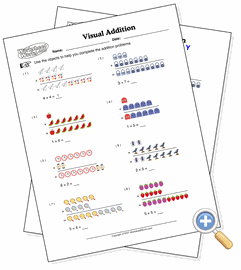# Visual AdditionDevelop addition skills using countable objects

These worksheets help to introduce basic addition by working with what the student already knows: how to count. Each addition operation is shown as both an equation of numerals and an equation of blocks. The blocks play the role of training wheels to let the student visually see how the two numerals can be used to produce a sum. There is also a worksheet generator for Visual Subtraction.